Learning Library

Subtraction Action! Ordering Numbers

With the help of these colorful critters, your second grader will practice double-digit subtraction and ordering numbers from least to greatest.

Click on a worksheet in the set below to see more info or download the PDF.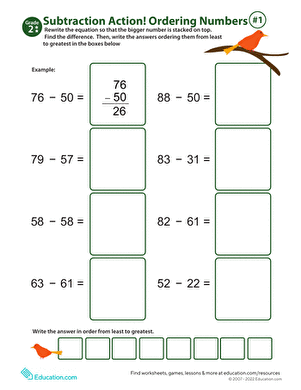Subtraction Action! Ordering Numbers #1

Maximize your second grader's at-home math practice with this fun worksheet, which combines subtraction and number ordering.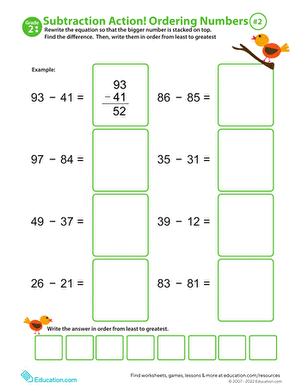Subtraction Action! Ordering Numbers #2

Incorporate both double-digit subtraction and ordering numbers into just one colorful worksheet!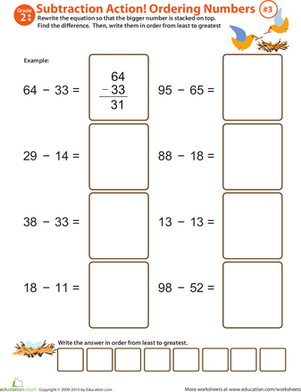Subtraction Action! Ordering Numbers #3

This colorful math worksheet has two advantages for your second grader! She'll boost her subtraction skills as she practices ordering numbers.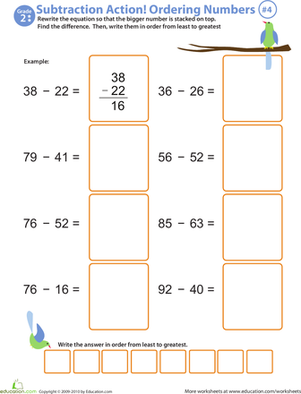Subtraction Action! Ordering Numbers #4

This worksheet is a double whammy; your second grader will practice double-digit subtraction and work on ordering numbers from least to greatest.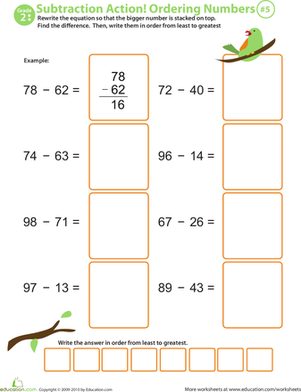Ordering Numbers

Let the pretty bird perched at the top of this worksheet be your second grader's guide through double-digit subtraction and ordering numbers.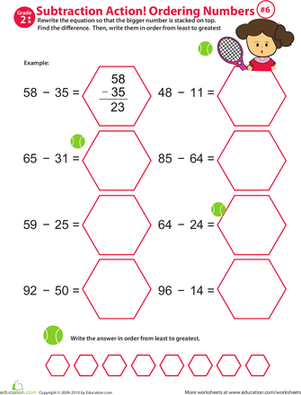Subtraction Action! Ordering Numbers #6

Your second grader will be challenged to solve double-digit subtraction and practice ordering numbers in this tennis-themed worksheet!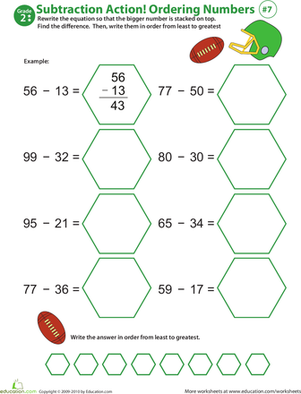Subtraction Action! Ordering Numbers #7

This colorful worksheet will help your second grader enhance her double-digit subtraction skills and ability to order numbers from least to greatest.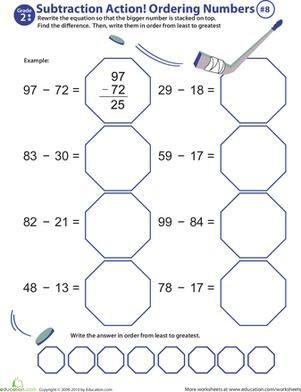Subtraction Action! Ordering Numbers #8

This colorful worksheet will give your second grader the boost she needs to become a subtraction know-it-all!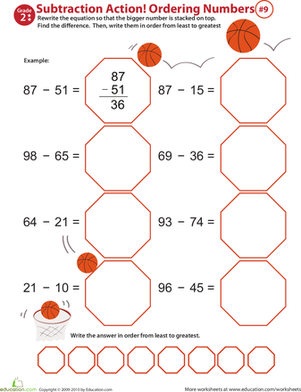Subtraction Action! Ordering Numbers #9

Spark your second grader's interest in subtraction with this fun, colorful worksheet!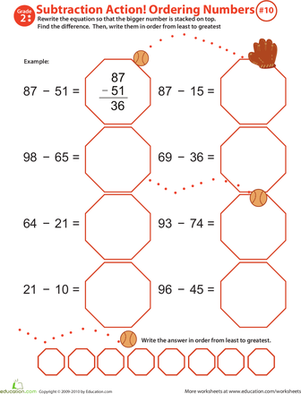Subtraction Action! Ordering Numbers #10

This delightful worksheet will maximize your second grader's at-home math time. He'll learn subtraction and practice ordering numbers from least to greatest.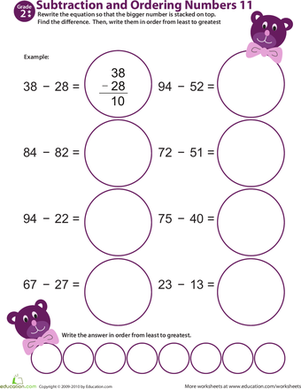Subtraction Action! Ordering Numbers #11

Get your child excited about subtraction with this 2nd grade math worksheet in which she'll practicing writing equations, subtracting, and ordering numbers.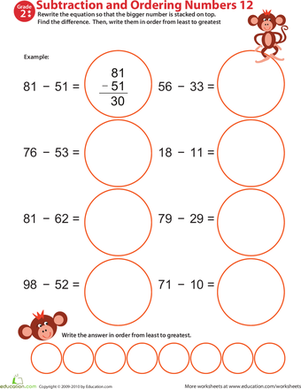Subtraction Action! Ordering Numbers #12

More than your average 2nd grade math worksheet, this printable makes practicing two-digit subtraction and place value fun!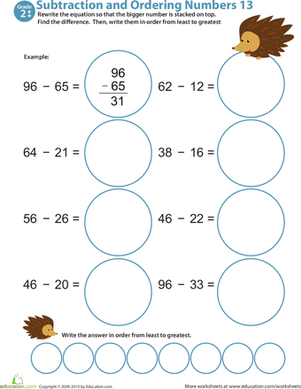Subtraction Action! Ordering Numbers #13

Help your child improve his subtraction facts fluency with this 2nd grade math worksheets featuring two-digit subtraction and number ordering.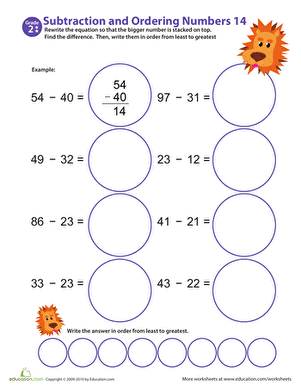Subtraction Action! Ordering Numbers #14

Help your child master two-digit subtraction with this cheerful math worksheet in which he'll rewrite equations, solve, then put his answers in numerical order.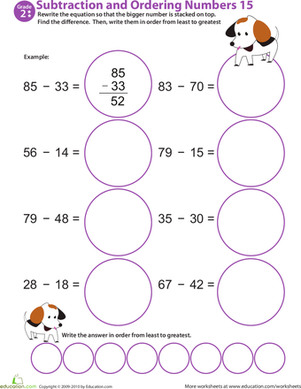Subtraction Action! Ordering Numbers #15

Download this 2nd grade math worksheet to give your child valuable at-home subtraction practice. Offers two-digit subtraction and a number ordering exercise.

Want to download this whole set as a single PDF?

Create new collection

0

New Collection>

0 items

What could we do to improve Education.com?

Please note: Use the Contact Us link at the bottom of our website for account-specific questions or issues.

What would make you love Education.com?